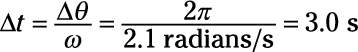##### Physics I Workbook For Dummies with Online Practice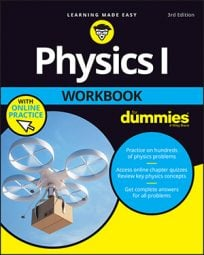There are analogs of every linear motion quantity (such as distance, velocity, and acceleration) in angular motion, and that’s one of the things that makes angular motion easier to work with after you have learned about linear motion. The velocity of an object in linear motion is shown in the following equation (this is actually a vector equation, of course, but you can look at this equation in scalar terms):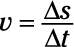What’s the analog of this equation in angular terms? That’s easy; you just substitute angle theta for the distance, so the angular velocity is theta/t. That means that angular velocity is the angle (in radians) that an object sweeps through per second. The symbol for angular velocity is omega, so you can write the equation for angular velocity this way: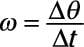The figure shows a line sweeping around in a circle. At a particular moment, it’s at angle theta, and if it took time t to get there, its angular velocity is omega = theta/t.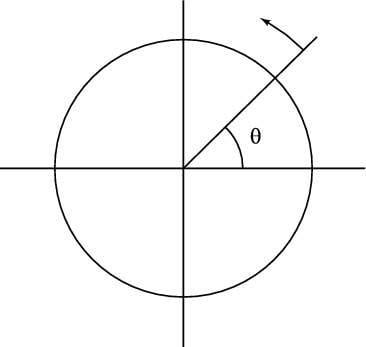So if the line completes a full circle in 1.0 s, its angular velocity is 2π/1.0 s = 2π radians/s (because there are 2π radians in a complete circle). Technically speaking, radian isn’t a physical unit of measure (it’s a ratio), so the angular velocity can also be written 2π s–1.

Given the angular velocity, you also can find the angle swept through in a number of seconds: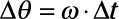## Sample question

1. The moon goes around Earth in about 27.3 days. What is its angular velocity?

1. Convert 27.3 days to seconds: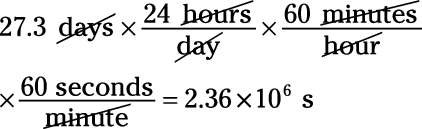2. Use the equation for angular velocity: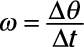3. Plug in the numbers: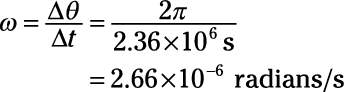## Practice questions

1. You have a toy plane on a string that goes around three complete circles in 9.0 s. What is its angular velocity?

2. You’re swinging a baseball bat around, getting ready for your shot at the ball. If you swing the bat in a half circle in 1.0 s, what is its angular velocity?

3. A satellite is orbiting Earth at 8.7 x 10–4 radians/s. How long will it take to circle the entire world?

4. A merry-go-round is spinning around at 2.1 radians/s. How long will it take to go in a complete circle?

Following are answers to the practice questions:

1. Use the equation for the angular velocity: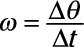2. Plug in the numbers: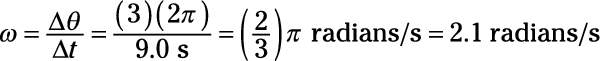1. Use the equation for the angular velocity:2. 2.Plug in the numbers: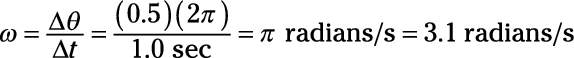3. 120 minutes2. Solve for Ä: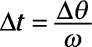3. 3.Plug in the numbers: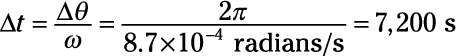4. 3.0 s2. Solve:3. Plug in the numbers: